0
127

# Data Interpretation Tables Questions [PDF] for MAH-CET

Question 1: In the table, the production and the sale (in 1000 tonnes) of a certain product of a company over5 years is given.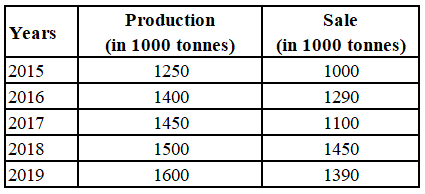In which year(s) the production increases by more than 10% of that in the previous year?

a) 2018, 2019

b) 2017, 2018

c) 2019

d) 2016

Solution:

Percentage increase in production of 2016 compared to 2015 = $\frac{150}{1250}\times$100 = 12%

Percentage increase in production of 2017 compared to 2016 = $\frac{50}{1400}\times$100 = 3.57%

Percentage increase in production of 2018 compared to 2017 = $\frac{50}{1450}\times$100 = 3.44%

Percentage increase in production of 2019 compared to 2018 = $\frac{100}{1500}\times$100 = 6.67%

$\therefore$  Only in 2016, the production increases by more than 10% of that in the previous year.

Hence, the correct answer is Option D

Question 2: Table shows District-wise data of number of primary school teachers posted in schools of a city.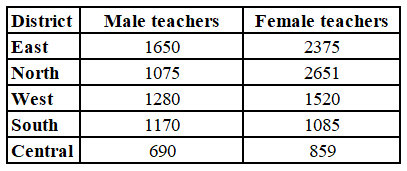What is the difference between the total number of male teachers in the districts East, North, West taken together and the total number of female teachers in the districts East and South?

a) 735

b) 771

c) 110

d) 545

Solution:

The total number of male teachers in the districts East, North, West taken together = 1650 + 1075 + 1280

= 4005

The total number of female teachers in the districts East and South = 2375 + 1085

= 3460

Required difference = 4005 – 3460

= 545

Hence, the correct answer is Option D

Question 3: Table shows the number of trees planted in 4 cities from 2016 to 2020.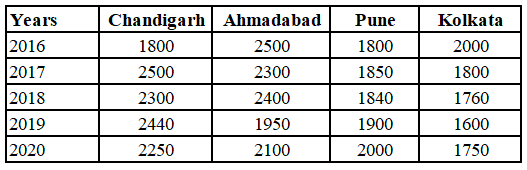In which city were maximum trees planted in 2016 and 2019 taken together?

a) Chandigarh

c) Pune

d) Kolkata

Solution:

Trees planted in Chandigarh in 2016 and 2019 together = 1800 + 2440 = 4240

Trees planted in Ahmedabad in 2016 and 2019 together = 2500 + 1950 = 4450

Trees planted in Pune in 2016 and 2019 together = 1800 + 1900 = 3700

Trees planted in Kolkata in 2016 and 2019 together = 2000 + 1600 = 3600

$\therefore$  In Ahmedabad, maximum trees planted in 2016 and 2019 taken together

Hence, the correct answer is Option B

Question 4: Study the following table and answer the question:
Percentage of marks obtained by six students in five subjects A, B, C, D & E.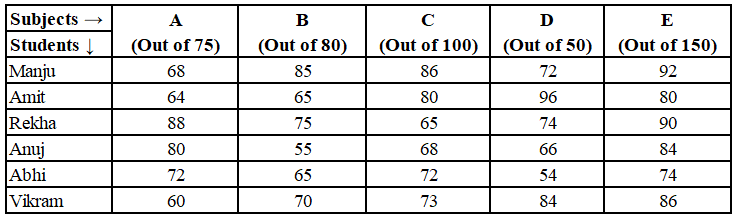The average marks of Manju, Rekha and Abhi in subject B are?

a) 54

b) 60

c) 56

d) 62

Solution:

Marks obtained by Majnu in subject B = $\frac{85}{100}\times$80 = 68

Marks obtained by Rekha in subject B = $\frac{75}{100}\times$80 = 60

Marks obtained by Abhi in subject B = $\frac{65}{100}\times$80 = 52

The average marks of Manju, Rekha and Abhi in subject B = $\frac{68+60+52}{3}$

= $\frac{180}{3}$

= 60

Hence, the correct answer is Option B

Question 5: The data given in the table shows the number of boys and girls enrolled in three different streams in a school over 5 years.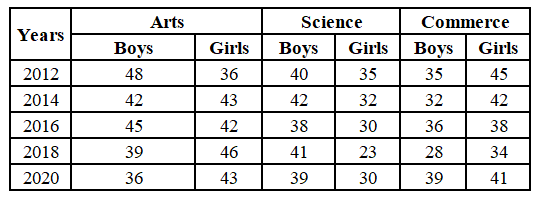What is the ratio of the total number of boys in the year 2014 to the total number of girls in the year 2020?

a) 58 : 57

b) 55 : 57

c) 58 : 53

d) 1 : 1

Solution:

Ratio of the total number of boys in the year 2014 to the total number of girls in the year 2020 = (42+42+32) : (43+30+41)

= 116 : 114

= 58 : 57

Hence, the correct answer is Option A

Take Free MAH-CET mock tests here

Question 6: Table shows income (in ₹) received by 4 employees of a company during the month of December 2020 and all their income sources.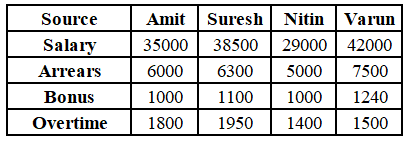What is the ratio of salary of Varun to his income other than salary?

a) 128 : 653

b) 653 : 128

c) 128 : 525

d) 525 : 128

Solution:

The ratio of salary of Varun to his income other than salary = 42000 : (7500+1240+1500)

= 42000 : 10240

= 525 : 128

Hence, the correct answer is Option D

Question 7: Table shows the number of trees planted in 4 cities from 2016 to 2020.What is the total number of trees planted in Chandigarh in 2017 and in Kolkata in 2020?

a) 4500

b) 4750

c) 4250

d) 3550

Solution:

From the table,

Number of trees planted in Chandigarh in 2017 = 2500

Number of trees planted in Kolkata in 2020 = 1750

The total number of trees planted in Chandigarh in 2017 and in Kolkata in 2020 = 2500 + 1750

= 4250

Hence, the correct answer is Option C

Question 8: Study the following table and answer the question:
Number of students Appeared (A) and Passed (P) in an annual examination from four schools Q, R, S & T in five years (2014 to 2018)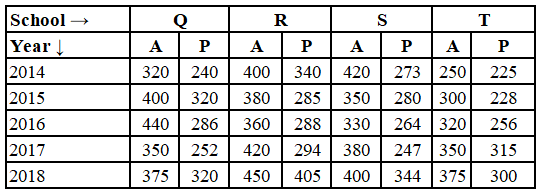The difference between the average number of students passed from school R in 2015 to 2017 and the number of students passed from school Q in 2015 is x. The value of x lies between:

a) 20 and 25

b) 35 and 40

c) 30 and 35

d) 25 and 30

Solution:

The difference between the average number of students passed from school R in 2015 to 2017 and the number of students passed from school Q in 2015 is x.

x = 320 – ($\frac{285+294}{2}$)

x = 320 – 289.5

x = 30.5

x lies between 30 and 35.

Hence, the correct answer is Option C

Question 9: Study the following table and answer the question:
Number of students Appeared (A) and Passed (P) in an annual examination from four schools Q, R, S & T in five years (2014 to 2018)The total number of students passed from school S in 2014 and 2017 is what percent of 90% of the total number of students appeared from school T in 2015, 2016 and 2017? (correct to one decimal place)

a) 53.9

b) 54.8

c) 59.6

d) 57.4

Solution:

The total number of students passed from school S in 2014 and 2017 = 273 + 247 = 520

90% of the total number of students appeared from school T in 2015, 2016 and 2017 = $\frac{90}{100}\left(300+320+350\right)$

= 873

Required percentage = $\frac{520}{873}\times100$

= 59.6(approximately)

Hence, the correct answer is Option C

Question 10: Study the following table and answer the question:
Number of students enrolled for Vocational Courses (VC) in institutes A, B, C, D, E & F.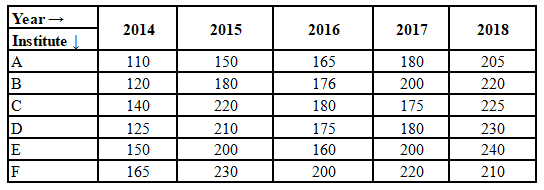The total number of students enrolled for VC in institutes D and F in 2014 is what percent of the total number of students enrolled in institutes A, B and C in 2018? (correct to one decimal point)

a) 43.2

b) 42.8

c) 43.8

d) 44.6

Solution:

The total number of students enrolled for VC in institutes D and F in 2014 = 125 +165 = 290

The total number of students enrolled for VC in institutes A, B and C in 2018 = 205 + 220 + 225 = 650

Required percentage = $\frac{290}{650}\times$100

= 44.61(approximately)

Hence, the correct answer is Option D

Question 11: Study the following table and answer the question:
Number of students enrolled for Vocational Courses (VC) in institutes A, B, C, D, E & F.The difference between the average number of students enrolled for VC in institute F in 2015, 2017 and 2018 and the average number of students enrolled in all the six institutes in 2014, is:

a) 89

b) 88

c) 82

d) 85

Solution:

The average number of students enrolled for VC in institute F in 2015, 2017 and 2018 = $\frac{230+220+210}{3}$ = 220

The average number of students enrolled for VC in all the six institutes in 2014 = $\frac{110+120+140+125+150+165}{6}$ = $\frac{810}{6}$ = 135

Required difference = 220 – 135 = 85

Hence, the correct answer is Option D

Question 12: Study the following table and answer the question:
Percentage of marks obtained by six students A, B, C, D, E and F in five subjects.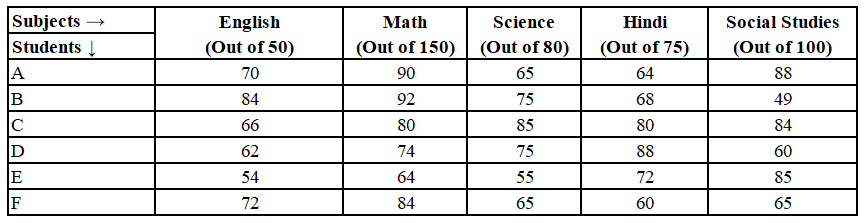The total marks obtained by student F in English, Science and Hindi is approximately what percent of the total marks obtained by student A in English, Mathematics, Science and Hindi? (Correct to one decimal place)

a) 50.2

b) 49.3

c) 48.4

d) 45.5

Solution:

The total marks obtained by student F in English, Science and Hindi = $\frac{72}{100}\times50+\frac{60}{100}\times80+\frac{60}{100}\times75$ = 36 + 52 + 45 = 133

The total marks obtained by student A in English, Mathematics, Science and Hindi = $\frac{70}{100}\times50+\frac{90}{100}\times150+\frac{60}{100}\times80+\frac{64}{100}\times75$ = 35 + 135 + 52 + 48 = 270

Required percentage = $\frac{133}{270}\times100$

= 49.259%

= 49.3% (approximately)

Hence, the correct answer is Option B

Question 13: Table shows District-wise data of number of primary school teachers posted in schools ofa city.
Study the table and answer the question.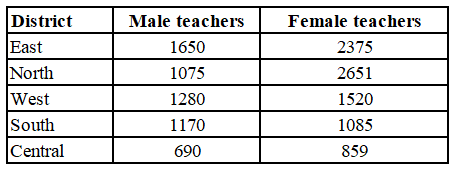In which district(s) is the number of female teachers Exceed the number of male teachers by more than 500?

a) West and South

b) East and North

c) North and South

d) East and West

Solution:

Difference between number of female teachers and male teachers respectively in East district = 2375 – 1650 = 725

Difference between number of female teachers and male teachers respectively in North district = 2651 – 1075 = 1576

Difference between number of female teachers and male teachers respectively in West district = 1520 – 1280 = 240

Difference between number of female teachers and male teachers respectively in Central district = 859 – 690 = 169

In East and North districts, number of female teachers Exceed the number of male teachers by more than 500.

Hence, the correct answer is Option B

Question 14: Study the following table and answer the question:
Percentage of marks obtained by six students A, B, C, D, E and in five subjects.What are the average marks of students B, C, D and F in Math?

a) 125.5

b) 82.5

c) 123.75

d) 120.75

Solution:

The average marks of students B, C, D and F in Math = $\frac{\frac{92}{100}\times150+\frac{80}{100}\times150+\frac{74}{100}\times150+\frac{84}{100}\times150}{4}$

= $\frac{\frac{150}{100}\left[92+80+74+84\right]}{4}$

= $\frac{\frac{150}{100}\left[330\right]}{4}$

= $\frac{495}{4}$

= 123.75

Hence, the correct answer is Option C

Question 15: In the table, production and sale (in 1000 tonnes) of a certain product of a company over 5 years is given. Study the table and answer the question.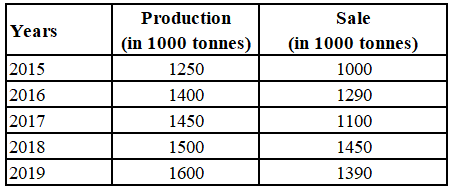In which year(s) sale is 80% or more but less than 90% of the production?

a) 2016, 2018

b) 2019

c) 2015, 2016

d) 2015, 2019

Solution:

In 2015, percentage of sales of the production = $\frac{1000}{1250}\times100$ = 80%

In 2016, percentage of sales of the production = $\frac{1290}{1400}\times100$ = 92.14%

In 2017, percentage of sales of the production = $\frac{1100}{1450}\times100$ = 75.86%

In 2018, percentage of sales of the production = $\frac{1450}{1500}\times100$ = 96.67%

In 2019, percentage of sales of the production = $\frac{1390}{1600}\times100$ = 86.875%

$\therefore$  In 2015 and 2019, sale is 80% or more but less than 90% of the production.

Hence, the correct answer is Option D

Question 16: The table shows the daily income (in ₹) of 50 persons.
Study the table and answer the question:What is the percentage of persons earning ₹250 or more?

a) 52

b) 68

c) 32

d) 48

Solution:

Number of persons earning less than ₹200 = 12

Number of persons earning between ₹200 and ₹250 = 26 – 12 = 14

Number of persons earning between ₹250 and ₹300 = 34 – 26 = 8

Number of persons earning between ₹300 and ₹350 = 40 – 34 = 6

Number of persons earning between ₹350 and ₹400 = 50 – 40 = 10

Percentage of persons earning ₹250 or more = $\frac{8+6+10}{50}\times100$ = 48%

Hence, the correct answer is Option D

Question 17: Study the following table and answer the question:
Percentage of marks obtained by six students in five subjects A, B, C, D & E.The total marks obtained by Amit in subjects A, B and C is what percent less than the total marks obtained by Vikram in subjects B, C, D and E?

a) 42

b) 35

c) 40

d) 38

Solution:

Total marks obtained by Amit in subjects A, B and C = $\frac{64}{100}\times75+\frac{65}{100}\times80+\frac{80}{100}\times100$ = 48 + 52 + 80 = 180

Total marks obtained by Vikram in subjects B, C, D and E = $\frac{70}{100}\times80+\frac{73}{100}\times100+\frac{84}{100}\times50+\frac{86}{100}\times150$ = 56 + 73 + 42 + 129 = 300

Required percentage = $\frac{300-180}{300}\times100$ = 40%

Hence, the correct answer is Option C

Question 18: The data given in the table shows the number of students studying in 4 different disciplines in 5 institutes. Study the table and answer the question: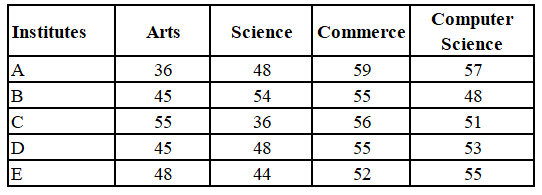What is the ratio of number of students studying Science in institutes C and D taken together to the number of students studying Computer Science in institutes A and E taken together?

a) 41:56

b) 43:56

c) 42:55

d) 3:4

Solution:

Number of students studying Science in institutes C and D taken together = 36 + 48 = 84

Number of students studying Computer Science in institutes A and E taken together = 57 + 55 = 112

Required ratio = 84 : 112

= 3 : 4

Hence, the correct answer is Option D

Question 19: Study the following table and answer the question:
Percentage of marks obtained by six students in five subjects A, B, C, D & E.The total marks obtained by Anuj in all the five subjects are ?

a) 331

b) 324

c) 303

d) 328

Solution:

The total marks obtained by Anuj in all the five subjects = $\frac{80}{100}\times75+\frac{55}{100}\times80+\frac{68}{100}\times100+\frac{66}{100}\times50+\frac{84}{100}\times150$

= 60 + 44 + 68 + 33 + 126

= 331

Hence, the correct answer is Option A

Question 20: The data given in the table shows the number of students studying in four different disciplines in 5 institutes. Study the table and answer the question.Number of students studying Computer Science in the institutes A and C taken together is what percent of the number of students studying Arts in the institutes B and D taken together?

a) 108

b) 200

c) 120

d) 83.3

Required percentage = $\frac{108}{90}\times100$ = 120%